# Division - Sharing Equally: Grade 3

##By university of Houston mathematics education

Use multiplication and division within 100 to solve word problems in situations involving equal groups, arrays, and measurement quantities, e.g., by using drawings and equations with a symbol for the unknown number to represent the problem.# Division - Sharing Equally: Grade 3

##By university of Houston mathematics education

Use multiplication and division within 100 to solve word problems in situations involving equal groups, arrays, and measurement quantities, e.g., by using drawings and equations with a symbol for the unknown number to represent the problem.# Fractions are Division

##By mathantics

A fraction is just a division problem written like a number. This video also reviews the parts of a fraction and introduces the idea of decimals.# Long Division

##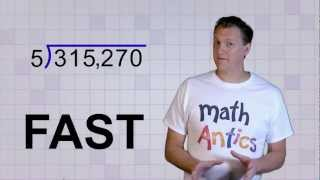By mathantics

Long Division# Introduction to Division

##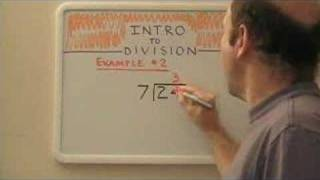By northstar15

Learn the basics of division including vocabulary and how to solve simple division problems. A teacher is using a whiteboard to explain the process as well as the vocabulary. He demonstrates two ways to write these division problems. Sound quality is poor.# One-step equations with multiplication and division

##By Khan Academy

Let's get a conceptual understanding of why one needs to divide both sides of an equation to solve for a variable.# One-step equations with multiplication and division

##By Khan Academy

This equation can be simplified through a single step to solve for the variable. Can you help?# One-step equations with multiplication and division

##By Khan Academy

Let's ease into this, shall we? Here's an introduction to basic algebraic equations of the form ax=b. Remember that you can check to see if you have the right answer by substituting it for the variable!# One-step equations with multiplication and division

##By Khan Academy

Remember that what you do to one side, you have to do to the other. Will you multiply or divide both sides to dump the fraction, x/a? Let's do it together.# Understanding fractions as division

##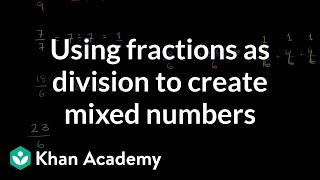By Khan Academy

Understand the relationship between fractions, division, and mixed numbers.# Dividing / Division of Integers

##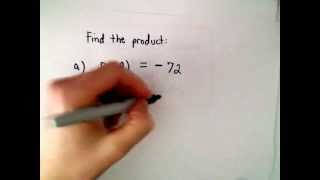By PatrickJMT

This video reviews the rule of signs. It then solves several multiplication and division problems using positive and negative integers.# Grade 6 Math - Division of Fractions 1

##By Lumos Learning

Using the Lumos Study Programs, parents and educators can reinforce the classroom learning experience for children and help them succeed at school and on the standardized tests. Lumos books, dvd, eLearning and tutoring are used by leading schools, libraries and thousands of parents to supplement classroom learning and improve student achievement in the standardized tests.# Introduction to long division

##By Khan Academy

Dividing into larger numbers. Introduction to long division without remainders# Understanding fractions as division

##By Khan Academy

Understand 3/2 on the number line by thinking about it as a mixed number.# Understanding fractions as division

##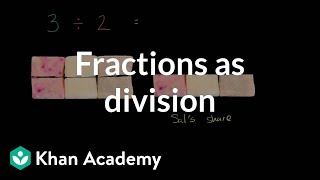By Khan Academy

Learn how 3 ï¿½ï¿½ï¿½ï¿½ï¿½ï¿½ï¿½ï¿½ï¿½ï¿½ï¿½ï¿½ 2 is the same as 3 ï¿½ï¿½ï¿½ï¿½ï¿½ï¿½ï¿½ï¿½ï¿½ï¿½ï¿½ï¿½ï¿½ï¿½ï¿½ï¿½ï¿½ï¿½ï¿½ï¿½ï¿½ 1/2, which is the same as 3/2.# Video for Lesson 11: Basic Division

##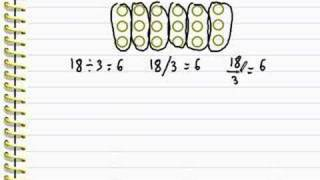By mathwithlarry

This is a video for basic division. Division is nothing more than repeated subtraction and multiplication is repeated addition. Examples are solved and explained on a piece of paper. He explains that division is the opposite of multiplication in his example.# Division Word Problems

##By TeacherTube Math

This is an explanation of how to solve a division word problem. She talks about writing down key words the steps to solving a word problem and checking your answer. Note that there is no actual word problem example in this video.# Dividing numbers: long division with remainders | Arithmetic | Khan Academy

##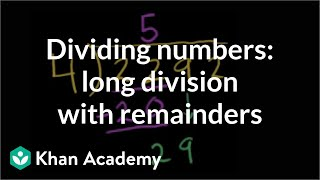By Khan Academy

Here we go with more long division practice. Ever wonder why we call it long division? What's long about it, anyway?# Expressing division in multiple ways

##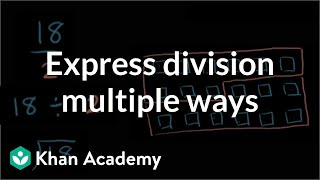By Khan,Academy

This word problem is solved by showing 4 different ways to divide 18 by 2.# Grade 4 Math - Division 7

##By Lumos Learning

Using the Lumos Study Programs, parents and educators can reinforce the classroom learning experience for children and help them succeed at school and on the standardized tests. Lumos books, dvd, eLearning and tutoring are used by leading schools, libraries and thousands of parents to supplement classroom learning and improve student achievement in the standardized tests.